• 31
•
• 2
•
•
•
•
•
33
Shares

# DIELECTRIC AND POLARIZATION

In this article we are going to talk about the concept of dielectric and polarization and related topics in detail, so stay with us till end…

## DIELECTRIC

Dielectrics are those substance which are non conducting. These are insulators, which does not allow electric current to pass through them. They are bad conductor of electric as well as heat.

Dielectrics materials can be made to hold an electrostatic charges with minimum dissipation of energy in form of heat. It dissipate minimum energy because it is a bad conductor of heat. Some examples of dielectrics are – Mica, plastic, glass, porcelain and various metals oxides.

## CLASSIFICATION OF DIELECTRICS

Dielectrics are classified into two types i.e

• Polar molecules
• Non polar molecules

### POLAR MOLECULES

Polar molecules are those type of dielectrics in which centre of gravity of positive charge and negative charge does not concide. The reason behind the molecules do not concide with each other is due to their asymmetrical shape. Some molecules which is polar dielectrics are :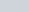,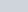,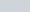etc.

We know that polar dielectric molecules do not concide with each other then the  seperation between the charges create a electric dipole moment in each molecules.

But this electric dipole moment be in random direction when there is no any external electric field is present. This random direction cancels the electric dipole moment of each molecules and net electric dipole moment became zero.

But if there is any external electric field is present then all the molecules aligned in the direction of electric field. See figure below:

### NON POLAR MOLECULES

Non polar molecules are those dielectric molecules whose centre of gravity concide with each other, means centre of positive charge and negative charge concide with each other.

There is no seperation distance between the electric charges that is why there is no any permanent electric dipole moment present in the molecules. Some examples are :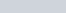etc.

## INDUCED ELECTRIC DIPOLE MOMENT

This type of induction happen in non polar molecules. When a non polar molecule is present in the external electric field then the positive charge particle i.e proton are pulled in the direction of electric field but the negative charge are pulled out in the opposite direction of electric field.

Due to the presence of external electric field this process continues unless the internal forces balance them. This process creates a seperation distance between the electric charges and this seperation distance creates a electric dipole moment in the molecules.

If dipole moment is created due to the presence of external electric field then the this type of electric dipole moment is called induced electric dipole moment. This induced electric dipole moment remains present in the molecules until external electric field is present, whenever the electric field absent then this type induction get disappeared.

## POLARIZABILITY

It is the tendency of the matter or molecules to acquire an electric dipole moment in proportion to that applied electric field when the molecules is placed in the external electric field.

## POLARIZATION DENSITY

When an external electric field is applied to a dielectric material it causes a displacement of charge particles i.e displacement of positive and negative charges. These charges which are bound to molecules and are not free to move around the material.

Positive charges are displaced in the direction of the electric field and negatively charged particles are displaced in the opposite of the direction of the electric field.  In this the neutrality of the molecules do not distrub and a electric dipole moment form in the molecules.

For a certain volume element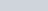in the material, which carries a dipole moment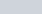, we define the polarization density P: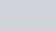In general, the dipole momentchanges from point to point within the dielectric. Hence, the polarization density P of a dielectric inside an infinitesimal volume dV with an infinitesimal dipole moment dp is given as: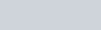The net charge appearing as a result of polarization is called bound charge and denoted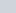.

Polarization density describes how a material responds to an applied electric field as well as the way the material changes the electric field, and it can be used to calculate the forces that result from those interactions

## ELECTRIC POLARIZATION

When a dielectric material is placed in an external electric field. It’s molecules gain electric dipole moment and the dielectric is said to be polarized. The electric dipole moment induced per unit volume of the dielectric material is called the electric polarization of the dielectric. Electric polarization is denoted by P.

## DIELECTRIC CONSTANT

It is the ratio of the strength of applied electric field to the strength of the reduced value of the electric field when a dielectric material is placed between the parallel plates of the capacitor.

It is also known as relative permittivity or specific inductive capacity and it is denoted by K or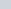. The dielectric constant of a dielectric medium is given as: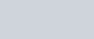## DIELECTRIC STRENGTH

Dielectric strength for a pure electrically insulating material is the maximum electric field that the material can withstand under ideal conditions without undergoing electrical breakdown and becoming electrically conductive.

In other words it is the strength of the dielectric material in which it can withstand in the maximum electric field without failure of its insulating property.

## ELECTRIC SUSCEPTIBILITY

It is the dimensionless proportionality constant which indicates the degree of polarization of dielectric material when it is placed in the exposure of electric field.

If the electric susceptibility of a material is higher then the material has higher ability to polarize in response to the electric field and and thereby reduce the total electric field inside the material. It influences the electric permittivity of the material.

### MATHEMATICAL EXPRESSION FOR ELECTRIC SUSCEPTIBILITY

Electric susceptibility can be defined as the relationship between the induced dielectric polarization density P and the electric field E, which is given as –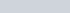Where

• is the induced dielectric polarization density.
•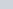is the electric permittivity of free space.
•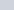is the electric susceptibility
• E is the electric field

Electric susceptibility is related to the relative permittivity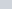(dielectric constant)  by –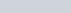So in case of vaccum= 0.